# Move the Matches Puzzles Episode 12 Levels 61-80 Walkthrough

Walkthrough for all the solutions and answers to the game Move the Matches Puzzles by K-Lab. Get the app on the Google Play Store to play along if you do not already have it. This is for episode 12, levels 61 through 80.

For the walkthrough (or answer) images, we will be showing the final image and answer of the Matches Puzzle. This will allow you to see where everything goes, and to know for sure that the puzzle works.  These puzzles have been recently updated, so other walkthroughs do not have the updated puzzles, but we try to always stay up to date with every walkthrough and answer!

### Level 61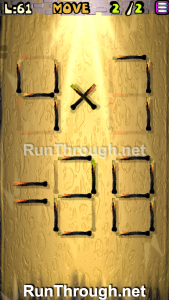Instructions: Move 2 matches to correct the equation

Solution: 4×7=28

### Level 62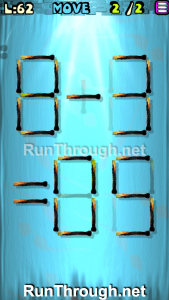Instructions: Move 2 matches to correct the equation

Solution: 8-3=05

### Level 63Instructions: Move 1 match to correct the equation

Solution: 7-4=3

### Level 64Instructions: Move 1 match to correct the equation

Solution: 11-9=2

### Level 65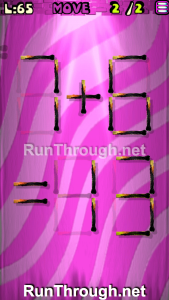Instructions: Move 2 matches to correct the equation

Solution: 7+6=13

### Level 66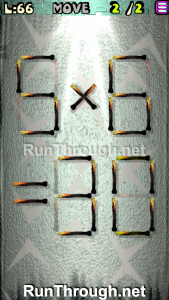Instructions: Move 2 matches to correct the equation

Solution: 5×6=30

### Level 67Instructions: Move 1 match to correct the equation

Solution: 6+2=8

### Level 68Instructions: Move 2 matches to correct the equation

Solution: 8×9=72

### Level 69Instructions: Move 1 match to correct the equation

Solution: 6×3=18

### Level 70Instructions: Move 4 matches to get 3 squares

### Level 71Instructions: Move 3 matches to correct the equation

Solution: 0/4=00

### Level 72Instructions: Move 2 matches to correct the equation

Solution: 8×4=32

### Level 73Instructions: Move 3 matches to correct the equation

Solution: 9/9=01

### Level 74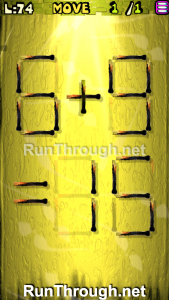Instructions: Move 1 match to correct the equation

Solution: 6+9=15

### Level 75Instructions: Move 1 match to correct the equation

Solution: 2+8=10

### Level 76Instructions: Remove 4 matches to correct the equation

Solution: 9×9=81

### Level 77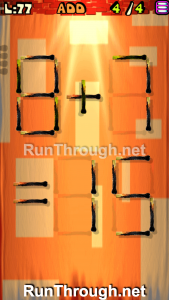Instructions: Add 4 matches to correct the equation

Solution: 8+7=15

### Level 78Instructions: Move 2 matches to correct the equation

Solution: 11-5=06

### Level 79Instructions: Move 2 matches to correct the equation

Solution: 9-0=09

### Level 80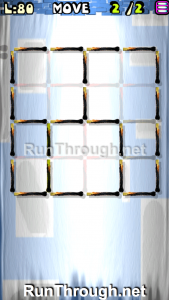Instructions: Move 2 matches